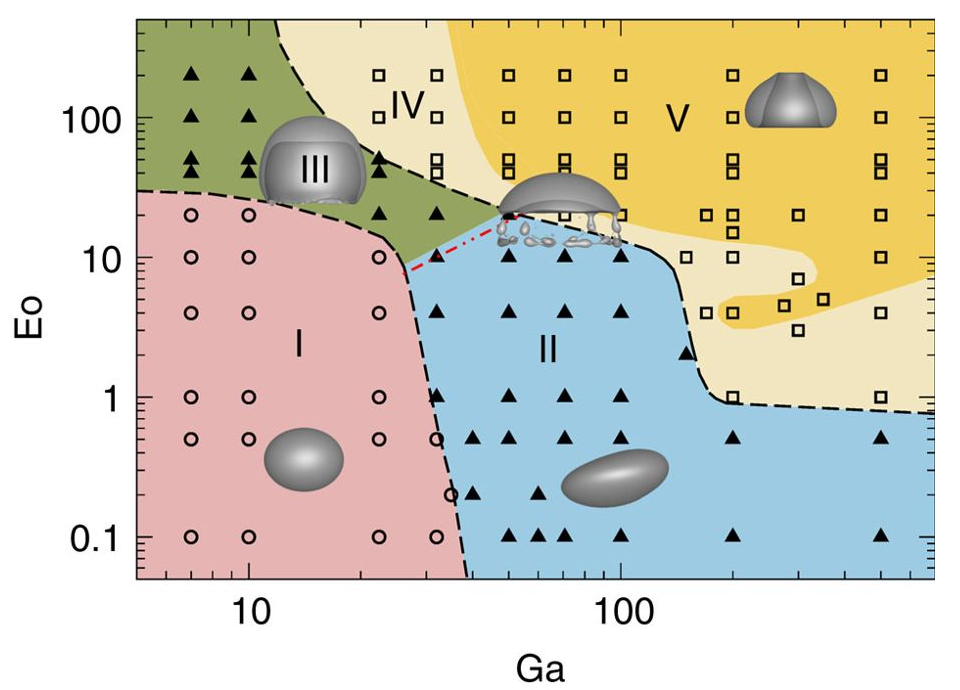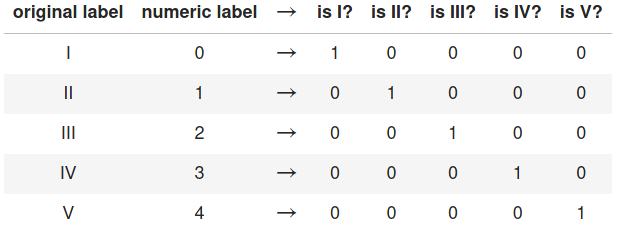### An introduction to supervised learning by example: path regime classification

Andre Weiner, Flow Modeling and Control Group
Technical University of Braunschweig, Institute of Fluid Mechanics

## difference between

• supervised ML,
• unsupervised ML,
• reinforcement learning?

## Deep learning?

1. synonym for ML
2. algorithm to find cats in images/videos
3. magical black box problem solver
4. none of the above

## (Deep) neural networks are used to solve

1. supervised ML problems
2. unsupervised ML problem
3. reinforcement learning problems
4. all of the above

## Python?

1. no idea
2. I know what it is but I've never used it
3. basic knowledge/experience

## Jupyter notebooks?

1. no idea
2. I know what it is but I've never used it
3. basic knowledge/experience

## Outline

1. ML terminology and notation
2. Path regime classification
3. Learning resources

Goal: understand when to use supervised ML

## ML terminology and notation

Just enough to get you started ...

## Features and Labels

Feature 1 $Re$ Feature 2 $\alpha$ ... Label 1 $c_d$ Label 2 regime
334 2 ... 0.123 laminar
334 4 ... 0.284 laminar
12004 2 ... 0.573 turbulent
12004 4 ... 0.834 turbulent
... ... ... ... ...

Image source: Kitware Inc., Flickr

## Supervised ML

Learning based on features and labels## Classification or regression?

Creating a transport model $\mu (T)$ based on experimental data ...

1. regression
2. classification
3. could be both

Creating a wall function to for the turbulent viscosity in RANS simulations ...

1. regression
2. classification
3. could be both

Impact behavior of a droplet on a surface ...

1. regression
2. classification
3. could be both

Predict the stability regime of a rising bubble ...

1. regression
2. classification
3. could be both

## Feature and label vectors

$N$ samples of $N_f$ features and $N_l$ labels

$i$ $x_{1}$ ... $x_{N_f}$ $y_{1}$ ... $y_{N_l}$
1 0.1 ... 0.6 0.5 ... 0.2
... ... ... ... ... ... ...
$N$ 1.0 ... 0.7 0.4 ... 0.2

ML models often map multiple inputs to multiple outputs!

## Feature vector

$$\mathrm{x} = \left[x_{1}, x_{2}, ..., x_{N_f}\right]^T$$ $\mathrm{x}$ - column vector of length $N_f$

$$\mathrm{X} = \left[\mathrm{x}_{1}, \mathrm{x}_{2}, ..., \mathrm{x}_{N_f}\right]$$ $\mathrm{X}$ - matrix with $N_s$ rows and $N_f$ columns

## Label vector

$$\mathrm{y} = \left[y_{1}, y_{2}, ..., y_{N_l}\right]^T$$ $\mathrm{y}$ - column vector of length $N_l$

$$\mathrm{Y} = \left[\mathrm{y}_{1}, \mathrm{y}_{2}, ..., \mathrm{y}_{N_l}\right]$$ $\mathrm{Y}$ - matrix with $N_s$ rows and $N_l$ columns

In the artificial dataset from before ($Re$, $\alpha$, $c_d$, regime), what is the value of $N_f$ if we use all available features?

1. 1
2. 2
3. 4

In the artificial dataset from before ($Re$, $\alpha$, $c_d$, regime), what is the value of $N_l$?

1. 1
2. 2
3. problem dependent

## ML model and prediction

$$f_\mathrm{w} : \mathbb{R}^{N_f} \rightarrow \mathbb{R}^{N_l}$$ $f_\mathrm{w}$ - ML model with weights $\mathrm{w}$ mapping from the feature space $\mathbb{R}^{N_f}$ to the label space $\mathbb{R}^{N_l}$ $$\hat{\mathrm{y}} = f_\mathrm{w}(x_1, x_2, ..., x_{N_f})$$ $\hat{\mathrm{y}}$ - (model) prediction

## Path regime classification

water/air: $d_{eq}=3~mm$
water/air: $d_{eq}=5~mm$Source: M. K. Tripathi et al. 2015, figure 1.

## Potential issues ...

assuming the decision boundary were created manually, e.g., using a graphical tool (Gimp, Inkscape, Photoshop, ...)1. easy
2. hard
3. impossible

1. easy
2. hard
3. impossible

## Data visualization## Feature distribution## What was different in the publication?

1. scaling to range $0...1$
2. normalization to zero mean and unity stdev
3. logarithmic axis

## Feature scaling## Scaled feature distribution## Issues with raw features?

1. numerical stability
2. high sensitivity to extreme data
3. low sensitivity to extreme data
4. unequal sensitivity to different features
5. all of the above

## Manual binary classification## Only regions I and II

$Ga^\prime = log(Ga)$, $Eo^\prime = log(Eo)$

$$z(Ga^\prime, Eo^\prime) = w_1Ga^\prime + w_2Eo^\prime + b$$

$$H(z (Ga^\prime, Eo^\prime)) = \left\{\begin{array}{lr} 0, & \text{if } z \leq 0\\ 1, & \text{if } z \gt 0 \end{array}\right.$$

Play with the sliders in the Jupyter notebook!## Compact notation

Linearly weighted inputs $$z_i=z(\mathrm{x}_i)=\sum\limits_{j=1}^{N_f}w_jx_{ij}$$

with $$\mathrm{x}_i = \left[ Ga^\prime_i, Eo^\prime_i, 1 \right],\quad \mathrm{w} = \left[ w_1, w_2, b \right]^T$$

## Binary encoding

True label: $$y_i = \left\{\begin{array}{lr} 0, & \text{for region I }\\ 1, & \text{for region II} \end{array}\right.$$

Predicted label: $$\hat{y}_i = H(z_i) = \left\{\begin{array}{lr} 0, & \text{if } z_i \leq 0\\ 1, & \text{if } z_i \gt 0 \end{array}\right.$$

## Loss function

Loss function $$L(\mathrm{w}) = \frac{1}{2}\sum\limits_{i=1}^N \left(y_i - \hat{y}_i(\mathrm{x}_i,\mathrm{w}) \right)^2$$

The term in parenthesis can take the values
$1$, $0$, or $-1$.

Simple update rule for the weights $$\mathrm{w}^{n+1} = \mathrm{w}^n - \eta \frac{\partial L(\mathrm{w})}{\partial \mathrm{w}} = \begin{pmatrix}w_1^n\\ w_2^n\\ b^n \end{pmatrix} + \eta \sum\limits_{i=1}^N \left(y_i - \hat{y}_i(\mathrm{x}_i,\mathrm{w}^n) \right) \begin{pmatrix}Ga^\prime_i\\ Eo^\prime_i\\ 1 \end{pmatrix}$$

$n$ - current iteration, $\eta$ - learning rate

performing gradient decent for some iterations ...the result ...## Issues with the perceptron algorithm?

Guaranteed convergence (zero loss)?

1. yes
2. no
3. data dependent

## Conditional probabilities

$$p(y=1 | \mathrm{x})$$

speak: probability $p$ of the event $y=1$ given $\mathrm{x}$

$$\hat{y} = f_\mathrm{w}(\mathrm{x}) = p(y=1 | \mathrm{x})$$

model predicts class probability instead of class!

What is the expected value of $p(y=1 | \mathrm{x})$ for points far in region II?

1. close to zero
3. close to one

What is the expected value of $p(y=1 | \mathrm{x})$ for points far in region I?

1. close to zero
3. close to one

What is the expected value of $p(y=1 | \mathrm{x})$ for points close to the decision boundary?

1. close to zero
3. close to one

What is the expected value of $p(y=0 | \mathrm{x})$ for points far in region I?

1. close to zero
3. close to one

How to convert $z(\mathrm{x})$ to a probability?

1. sinus: $\mathrm{sin}(z)$
2. hyperbolic tangents: $\mathrm{tanh}(z)$
3. sigmoid: $\sigma(z)$

sigmoid function $\sigma (z) = \frac{1}{1+e^{-z}}$based on the perceptron classifier's weights ...## Joint probabilities - likelihood function

$$l(\mathrm{w}) = \prod\limits_i^{N} p_i(y_i | \mathrm{x}_i, \mathrm{w})$$

principle of maximum likelihood

$$\mathrm{w}^* = \underset{\mathrm{w}}{\mathrm{argmax}}\ l(\mathrm{w}).$$

Which of the following is equivalent to $l(\mathrm{w}) = \prod\limits_i^{N} p_i(y_i | \mathrm{x}_i, \mathrm{w})$?

1. $$\prod\limits_i^{N} \left[ y_i^{\hat{y}_i} (1-y_i)^{(1-\hat{y}_i)}\right]$$
2. $$\prod\limits_i^{N} \left[ \hat{y}_i^{y_i} (1-\hat{y}_i)^{(1-y_i)}\right]$$

## Log-likelihood and binary cross entropy

$$\mathrm{log}(l(\mathrm{w})) = \sum\limits_{i=1}^N y_i \mathrm{ln}(\hat{y}_i) + (1-y_i) \mathrm{ln}(1-\hat{y}_i)$$

$$L(\mathrm{w}) = -\frac{1}{N}\sum\limits_{i=1}^N y_i \mathrm{ln}(\hat{y}_i) + (1-y_i) \mathrm{ln}(1-\hat{y}_i)$$

performing gradient decent for some iterations ...the result ...Next step: separating region I from region II and IIIFirst: two linear modelsRecipe to combine the two linear models:

1. compute weighted sum of individual models
2. convert the weighted sum to a probability using $\sigma$

combined linear models## One hot encodingWhat is the length of the label vector if we have 10 classes (regimes)?

1. 1
2. 2
3. 5
4. 10

What does the label vector look like if we have 4 classes and the true label is class 3 / regime 3?

1. $\mathrm{y}_i = \left[ 1, 0, 0, 0 \right]^T$
2. $\mathrm{y}_i = \left[ 0, 0, 1, 0 \right]^T$
3. $\mathrm{y}_i = \left[ 1, 0, 1, 0 \right]^T$
4. $\mathrm{y}_i = \left[ 0, 0, 3, 0 \right]^T$

Softmax function and categorical cross entropy for $K$ classes:

$$p(y_{j}=1 | \mathrm{x}) = \frac{e^{z_{j}}}{\sum_{j=0}^{K-1} e^{z_{j}}}$$

$$L(\mathrm{w}) = -\frac{1}{N} \sum\limits_{j=0}^{K-1}\sum\limits_{i=1}^{N} y_{ij} \mathrm{ln}\left( \hat{y}_{ij} \right)$$

## Implementation in PyTorch


class PyTorchClassifier(nn.Module):
'''Multi-layer perceptron with 3 hidden layers.
'''
def __init__(self, n_features=2, n_classes=5, n_neurons=60, activation=torch.sigmoid):
super().__init__()
self.activation = activation
self.layer_1 = nn.Linear(n_features, n_neurons)
self.layer_2 = nn.Linear(n_neurons, n_neurons)
self.layer_3 = nn.Linear(n_neurons, n_classes)

def forward(self, x):
x = self.activation(self.layer_1(x))
x = self.activation(self.layer_2(x))
return F.log_softmax(self.layer_3(x), dim=1)


Hurray!# THE END

### Thank you for you attention!

Where to go from here?